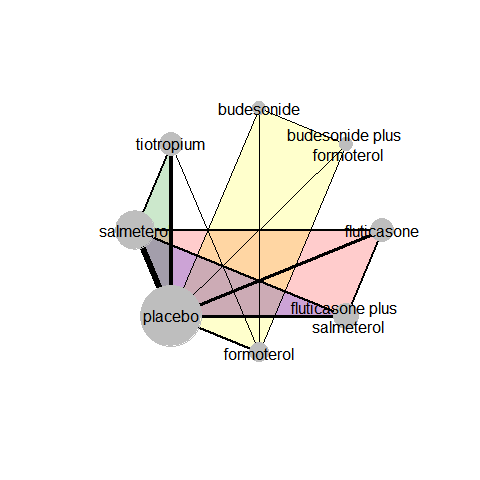# Perform network meta-analysis

## Introduction

This vignette illustrates how to perform a one-stage Bayesian random-effects network meta-analysis with consistency equation using the run_model function. This function includes arguments to handle aggregate missing participant outcome data (MOD) in each arm of every trial via the pattern-mixture model.

## Example on a binary outcome

We will use the network on pharmacologic interventions for chronic obstructive pulmonary disease (COPD) from the systematic review of Baker et al. (2009). This network comprises 21 trials comparing seven pharmacological interventions with each other and placebo. The exacerbation of COPD (harmful outcome) is the analysed binary outcome (see ?nma.baker2009).## Perform Bayesian random-effects network meta-analysis

### Run the model

run_model calls the jags function from the R2jags package to perform the Bayesian analysis using the BUGS code of Dias and colleagues (2013).

run_model(data = nma.baker2009,
measure = "OR",
heter_prior = list("halfnormal", 0, 1),
D = 0,
n_chains = 3,
n_iter = 10000,
n_burnin = 1000,
n_thin = 1)

With only the minimum required arguments, the function adjusts MOD under the missing-at-random assumption (MAR; by default) via the informative missingness odds ratio (IMOR) in the logarithmic scale (White et al. (2008)): The minimum required arguments of run_model include specifying:

• the dataset (a data-frame with one-trial-per-row format) in data (see ?data_preparation);
• the effect measure in measure (see ‘Arguments’ in ?run_model):
• the prior distribution for the heterogeneity parameter in heter_prior (see ?heterogeneity_param_prior);
• the direction of the outcome in D (here, D = 0 because the outcome is harmful; see, ‘Arguments’ in ?run_model)
• the number of chains in n_chains (see ‘Arguments’ in ?run_model – also for the subsequent arguments);
• the number of iterations in n_iter;
• the number of burn-in in n_burnin, and
• the thinning in n_thin.

### Using all arguments

Suppose we decide to use an empirically-based prior distribution for the between-trial variance that aligns with the outcome and interventions under investigation. We also consider a hierarchical structure for the prior normal distribution of the log IMOR that is specific to the interventions in the network (assumption = "HIE-ARM") (Turner et al., 2015a; Spineli, 2019). We still assume MAR on average with variance of log IMOR equal to 1 (var_misspar = 1) which is also the default argument. In this case, run_model must be specified as follows:

run_model(data = nma.baker2009,
measure = "OR",
model = "RE",
assumption = "HIE-ARM",
heter_prior = list("lognormal", -2.06, 0.438),
mean_misspar = c(0, 0),
var_misspar = 1,
D = 0,
n_chains = 3,
n_iter = 10000,
n_burnin = 1000,
n_thin = 1)

The argument model = "RE" refers to the random-effects model. For the fixed-effect model, use model = "FE".

heter_prior = list("lognormal", -2.06, 0.438) refers to ‘symptoms reflecting the continuation of condition’ for the ‘pharmacological versus placebo’ comparison-type as elicited by Turner and colleagues (2015b).

In the argument mean_misspar = c(0, 0), the first and second element of the vector refers to the mean log IMOR in the non-reference interventions and the reference intervention of the network, respectively – the latter is always the intervention with identifier equal to 1. Hence, for all non-reference interventions we can consider the same mean log IMOR. See ‘Details’ in ?missingness_param_prior

### The output

run_model returns a list of R2jags output on the summaries of the posterior distribution, and the Gelman-Rubin convergence diagnostic of the monitored parameters (see ‘Value’ in ?run_model). The output is used as an S3 object by other functions of the package to be processed further and provide an end-user-ready output. See, for example, the function ?league_heatmap that creates the league table with the effect sizes of all possible comparisons in the network.

### No or partially extracted missing participant outcome data

run_model can also handle a dataset where MOD have not be extracted or MOD have been extracted for some trials or trial-arms. For illustrative purposes, we removed the item m from nma.baker2009 to indicate that MOD were not extracted for this outcome:

                  study t1 t2 t3 t4 r1 r2 r3 r4  n1  n2 n3 n4
1 Llewellyn-Jones, 1996  1  4 NA NA  3  0 NA NA   8   8 NA NA
2        Paggiaro, 1998  1  4 NA NA 51 45 NA NA 139 142 NA NA
3          Mahler, 1999  1  7 NA NA 47 28 NA NA 143 135 NA NA
4        Casaburi, 2000  1  8 NA NA 41 45 NA NA 191 279 NA NA
5       van Noord, 2000  1  7 NA NA 18 11 NA NA  50  47 NA NA
6         Rennard, 2001  1  7 NA NA 41 38 NA NA 135 132 NA NA

Using the minimum required arguments, run_model will run and provide results.

run_model calls the data_preparation function. The latter creates a pseudo-data-frame for the item m (see ‘Value’ in ?data_preparation) that assigns NA to all trial-arms. data_preparation also creates the pseudo-data-frame I that has the same dimension with the other items in the dataset, and assigns the zero value to all trial-arms to indicate that no MOD have been extracted. Both pseudo-data-frames aim to retain in the dataset the trials without information on MOD; otherwise, these trials would have been excluded from the analysis. See ‘Details’ in ?data_preparation and ?run_model.